NEET  >  Test: Dual Nature of Matter & Heisenberg's Uncertainty Principle

# Test: Dual Nature of Matter & Heisenberg's Uncertainty Principle

Test Description

## 16 Questions MCQ Test Chemistry Class 11 | Test: Dual Nature of Matter & Heisenberg's Uncertainty Principle

Test: Dual Nature of Matter & Heisenberg's Uncertainty Principle for NEET 2022 is part of Chemistry Class 11 preparation. The Test: Dual Nature of Matter & Heisenberg's Uncertainty Principle questions and answers have been prepared according to the NEET exam syllabus.The Test: Dual Nature of Matter & Heisenberg's Uncertainty Principle MCQs are made for NEET 2022 Exam. Find important definitions, questions, notes, meanings, examples, exercises, MCQs and online tests for Test: Dual Nature of Matter & Heisenberg's Uncertainty Principle below.
Solutions of Test: Dual Nature of Matter & Heisenberg's Uncertainty Principle questions in English are available as part of our Chemistry Class 11 for NEET & Test: Dual Nature of Matter & Heisenberg's Uncertainty Principle solutions in Hindi for Chemistry Class 11 course. Download more important topics, notes, lectures and mock test series for NEET Exam by signing up for free. Attempt Test: Dual Nature of Matter & Heisenberg's Uncertainty Principle | 16 questions in 16 minutes | Mock test for NEET preparation | Free important questions MCQ to study Chemistry Class 11 for NEET Exam | Download free PDF with solutions
 1 Crore+ students have signed up on EduRev. Have you?
Test: Dual Nature of Matter & Heisenberg's Uncertainty Principle - Question 1

### Direction (Q. Nos. 1-10) This section contains 10 multiple choice questions. Each question has four choices (a), (b), (c) and (d), out of which ONLY ONE option is correct. Which of the following properties of atom could be explained correctly by Thomson Model of atom?

Detailed Solution for Test: Dual Nature of Matter & Heisenberg's Uncertainty Principle - Question 1

According to Thomson model of atom, the mass of the atom is assumed to be uniformly distributed over the atom. This model was able to explain the overall neutrality of the atom.

Test: Dual Nature of Matter & Heisenberg's Uncertainty Principle - Question 2

### The threshold frequency v0 for a metal is 7.0 x 1014 s-1. Radiation of frequency v = 1.0 x 1015 s-1 hits the metal. Kinetic energy of the emitted electron is

Detailed Solution for Test: Dual Nature of Matter & Heisenberg's Uncertainty Principle - Question 2

By photoelectric effect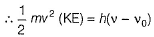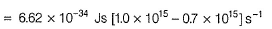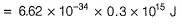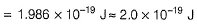Test: Dual Nature of Matter & Heisenberg's Uncertainty Principle - Question 3

### Which of the following rules/principles is responsible to rule out the existence of definite paths or trajectories of electrons?

Detailed Solution for Test: Dual Nature of Matter & Heisenberg's Uncertainty Principle - Question 3

By Heisenberg’s uncertainty principle, it is impossible to determine position and momentum simultaneously with same accuracy. Thus, existence of definite path is ruled out.

Test: Dual Nature of Matter & Heisenberg's Uncertainty Principle - Question 4

In an atom, an electron is moving with a speed of 600 ms-1 with an accuracy of 0.005%. Certainty with which the position of the electron can be located is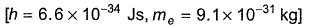[AIEEE 2009]

Detailed Solution for Test: Dual Nature of Matter & Heisenberg's Uncertainty Principle - Question 4

By Heisenberg’s uncertainty principle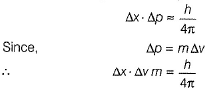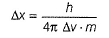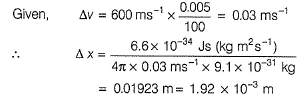Test: Dual Nature of Matter & Heisenberg's Uncertainty Principle - Question 5

The wavelength (in nanometer) associated with a proton (mass = 1.67 x 10-27 kg atom-1) at the velocity of 1.0 x 10-3 ms-1 is

Detailed Solution for Test: Dual Nature of Matter & Heisenberg's Uncertainty Principle - Question 5

By de-Broglie’s equation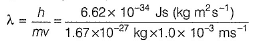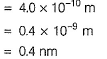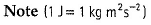Test: Dual Nature of Matter & Heisenberg's Uncertainty Principle - Question 6

Statement I : It is impossible to determine position and momentum of the moving electron simultaneously with same accuracy.
Statement II : The path of an electron in an atom is clearly defined.

Detailed Solution for Test: Dual Nature of Matter & Heisenberg's Uncertainty Principle - Question 6

Statement 1 is uncertainty principle, according to which we can't locate a moving electron accurately at any instant, which implies there must me certain uncertainty in its path, as path basically gives us the position of electron at various instances.

Test: Dual Nature of Matter & Heisenberg's Uncertainty Principle - Question 7

If the photon of the wavelength 150 pm strikes an atom and one of its inner bound electrons is ejected out with a velocity of 1.5 x 107 ms-1, then binding energy by which electron is bound to nucleus is

Detailed Solution for Test: Dual Nature of Matter & Heisenberg's Uncertainty Principle - Question 7

Binding energy is the minimum energy to which electron is bound to nucleus or which is required to eject the electron.
Thus, binding energy = - threshold energy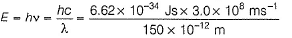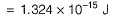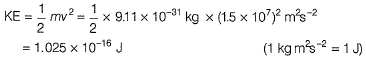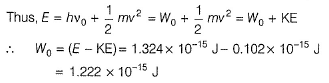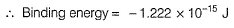Test: Dual Nature of Matter & Heisenberg's Uncertainty Principle - Question 8

The position of both, an electron and a helium atom is known within 1.0 mm. Further more the momentum of the electron is known within 5.0 x 10-26 kg ms-1. The minimum uncertainty in the measurement of the momentum of the helium atom is

Detailed Solution for Test: Dual Nature of Matter & Heisenberg's Uncertainty Principle - Question 8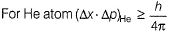and for electron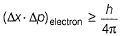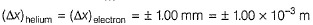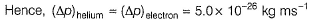Test: Dual Nature of Matter & Heisenberg's Uncertainty Principle - Question 9

The wavelength of a neutron with a translatory kinetic energy equal to kT at 300 K is

Detailed Solution for Test: Dual Nature of Matter & Heisenberg's Uncertainty Principle - Question 9

Kinetic energy = kT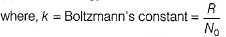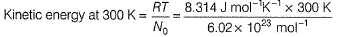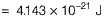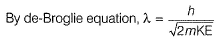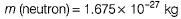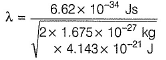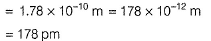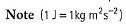Test: Dual Nature of Matter & Heisenberg's Uncertainty Principle - Question 10

Frequency of a matter wave is equal to

Detailed Solution for Test: Dual Nature of Matter & Heisenberg's Uncertainty Principle - Question 10

By de-Broglie equation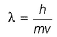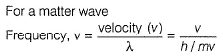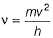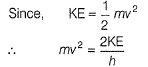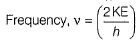Test: Dual Nature of Matter & Heisenberg's Uncertainty Principle - Question 11

Direction (Q. Nos. 11 and 12) This section contains a paragraph, wach describing  theory, experiments, data etc. three Questions related to paragraph have been  given.Each question have only one correct answer among the four given  ptions  (a),(b),(c),(d)

Photoelectric effect can be expressed in terms of the following graph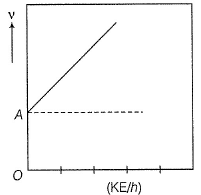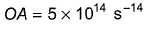Q. What is work function for this photoelectric emission of electrons?

Detailed Solution for Test: Dual Nature of Matter & Heisenberg's Uncertainty Principle - Question 11

Photoelectric effect is represented by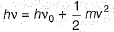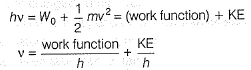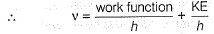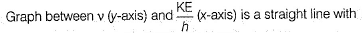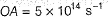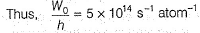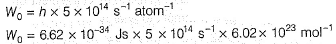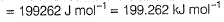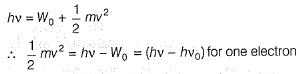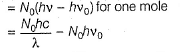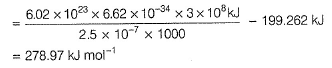Test: Dual Nature of Matter & Heisenberg's Uncertainty Principle - Question 12

Photoelectric effect can be expressed in terms of the following graphQ. Kinetic energy imparted to the moving electron at a wavelength of 2.5 x 10-7 m is

Detailed Solution for Test: Dual Nature of Matter & Heisenberg's Uncertainty Principle - Question 12

Photoelectric effect is represented byTest: Dual Nature of Matter & Heisenberg's Uncertainty Principle - Question 13

Direction (Q. Nos. 13) Choice the correct combination of elements and column I and coloumn II are given as option (a), (b), (c) and (d), out of which ONE option is correct.

Q.

Neon gas is generally used in sign boards. It emits radiations at wavelength 616 nm.
Match the values derived given in Column II with the corresponding parameter given in Column I.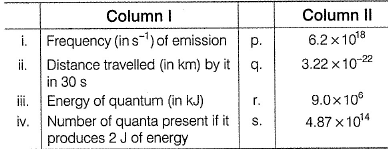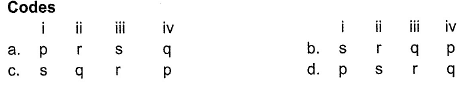Detailed Solution for Test: Dual Nature of Matter & Heisenberg's Uncertainty Principle - Question 13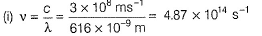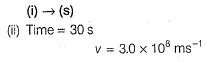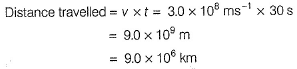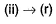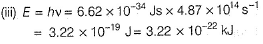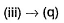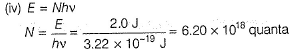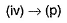*Answer can only contain numeric values
Test: Dual Nature of Matter & Heisenberg's Uncertainty Principle - Question 14

Direction (Q. Nos. 14 - 16) This section contains 2 questions. when worked out will result in an integer from 0 to 9 (both inclusive)

Q.

The work function (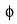) of some metals is listed below. The number of metals which will show photoelectric effect when light of 300 nm wavelength falls on the metal i s ........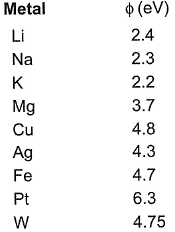Detailed Solution for Test: Dual Nature of Matter & Heisenberg's Uncertainty Principle - Question 14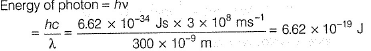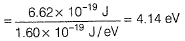Thus, any metal with energy less than 4.14 eV will emit photons thus, showing photoelectric effect. (Li, Na, K, Mg)

*Answer can only contain numeric values
Test: Dual Nature of Matter & Heisenberg's Uncertainty Principle - Question 15

If the uncertainties in the measurements of position and momentum are equal, calculate the uncertainty in the measurement of velocity (in ms-1) of particle of mass 1.21 x 10-18 kg

Detailed Solution for Test: Dual Nature of Matter & Heisenberg's Uncertainty Principle - Question 15

By Heisenberg’s uncertainty principle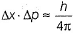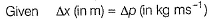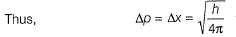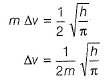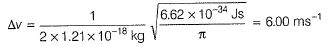*Answer can only contain numeric values
Test: Dual Nature of Matter & Heisenberg's Uncertainty Principle - Question 16

Wavelength associated with an electron having KE 3.0 x 10-25 J is x *10-7 m.

Q. What is the value of x?

Detailed Solution for Test: Dual Nature of Matter & Heisenberg's Uncertainty Principle - Question 16

By de-Broglie equation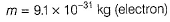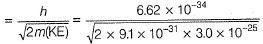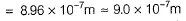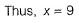## Chemistry Class 11

204 videos|320 docs|229 tests
 Use Code STAYHOME200 and get INR 200 additional OFF Use Coupon Code
Information about Test: Dual Nature of Matter & Heisenberg's Uncertainty Principle Page
In this test you can find the Exam questions for Test: Dual Nature of Matter & Heisenberg's Uncertainty Principle solved & explained in the simplest way possible. Besides giving Questions and answers for Test: Dual Nature of Matter & Heisenberg's Uncertainty Principle, EduRev gives you an ample number of Online tests for practice

## Chemistry Class 11

204 videos|320 docs|229 tests

### How to Prepare for NEET

Read our guide to prepare for NEET which is created by Toppers & the best Teachers